of a finite groupA representationofin a finite-dimensional vector space(cf. Representation of a group) such that in some basis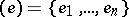ofthe matrix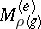of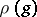, for any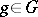, has only one non-zero element in each row and each column. Sometimes, by a monomial representation is meant the regular matrix representation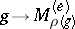(cf. also Regular representation). A monomial representation is a special case of an imprimitive representation (see Imprimitive group). Namely, the set of one-dimensional subspaces ingenerated by the vectorsis a system of imprimitivity for. Conversely, if for some representationofin a vector spacethere is a system of imprimitivity consisting of one-dimensional subspaces, thenis a monomial representation. Letbe any subgroup of; an example of a monomial representation is the representation ofinduced from a one-dimensional representation of(see Induced representation). Such a representation is also called an induced monomial representation (see ). Not every monomial representationis induced (however, ifis irreducible, then it will be induced). The definition of a monomial representation given above arose in the classical theory of representations of finite groups. Often, however, this definition can be modified — any representation of a groupinduced from a one-dimensional representation of some subgroupof it being called a monomial representation. In this form the definition of a monomial representation makes sense not just for finite groups and their finite-dimensional representations, but also, for example, for Lie groups and their representations in Hilbert spaces (the subgroupis then assumed to be closed). For a fairly large class of groups the construction of the monomial representations turns out to be sufficient for the description of all irreducible unitary representations. Namely, groups all irreducible unitary representations (cf. Irreducible representation; Unitary representation) of which are monomial are called monomial groups, or-groups. They include all finite nilpotent groups and all connected nilpotent Lie groups. All finite-groups, and also all monomial Lie groups, are solvable (see ).